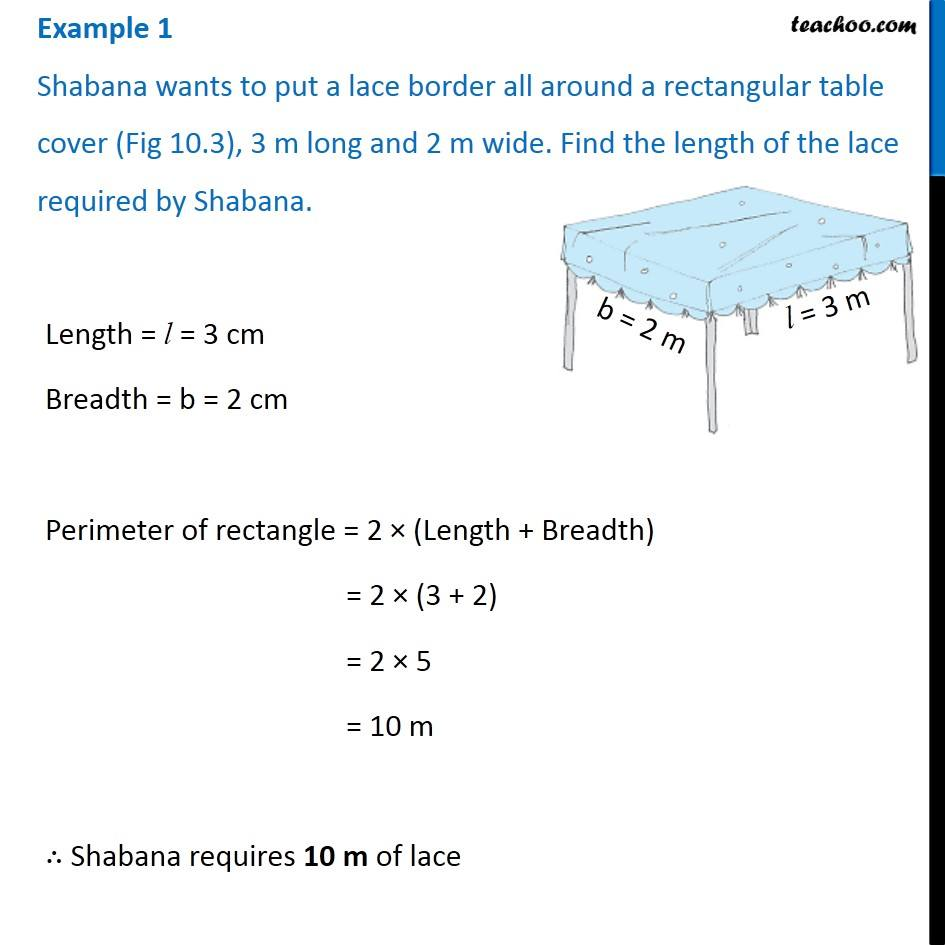Examples

Chapter 10 Class 6 Mensuration
Serial order wise### Transcript

Example 1 Shabana wants to put a lace border all around a rectangular table cover (Fig 10.3), 3 m long and 2 m wide. Find the length of the lace required by Shabana. Length = l = 3 cm Breadth = b = 2 cm Perimeter of rectangle = 2 × (Length + Breadth) = 2 × (3 + 2) = 2 × 5 = 10 m ∴ Shabana requires 10 m of lace